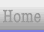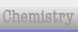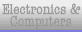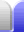# Polygons & Polyhedra

### Polygons

A polygon is a multi-sided shape. Simple examples are triangles and rectangles.

A regular polygon is a multi-sided shape where all the sides are the same length. A simple example would be an equilateral triangle or a square. We can also describe a polygon as convex if all the internal angles are less than 180°.

### Polyhedra

A polyhedron is a multi-faced object. Simple examples are boxes and prisms (triangular or rectangular).

A regular polyhedron is one where all the faces are the same regular polygon. There are just five convex regular polyhedra, known as the Platonic solids, after Greek philosopher Plato, although they were known of well before his time.

Here are the five Platonic solids.

 Tetrahedron or triagonal pyramid Hexahedron or cube Octahedron Dodecahedron Icosahedron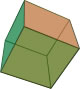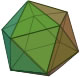4 faces equilateral triangles 6 faces squares 8 faces equilateral triangles 12 faces regular pentagons 20 faces equilateral triangles
 Swapping the faces and vertices (corners) of a solid makes its dual. In other words, putting a vertex of the new solid in the middle of each face of the original solid.The cube and the octahedron are the dual of each other (see the pic), and the dodecahedron and the icosahedron are the dual of each other. The tetrahedron is self-dual – if you join the points at the centre of each face you get another tetrahedron.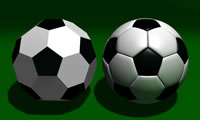A soccer ball is a truncated (chopped off) icosahedron, which basically means the points have been cut off an icosahedron leaving 20 hexagons and 12 pentagons. As well as graphite and diamond, carbon can also form a C60 molecule – buckminsterfullerene, also known as buckyballs. It's a truncated icosahedron and is naturally occuring in soot. It's the largest particle which has been shown to have displayed wave-particle duality.### Polyhedral DiceSince the faces of each Platonic solid have exactly the same area, we can use the Platonic solids as dice. The Platonic solids give us the standard six faced die, as well as dice with four, eight, twelve and twenty faces. We can also use non-Platonic solids as dice. A ten sided polyhedra (which has kite-shaped sides) is great for a die with ten faces. All the faces are the same shape and have the same area, but the faces are not regular polygons because their sides are not all the same length. In the picture at the right, there are two 10-sided dice, one with 0-10 and the other with 00-90. 24 sided dice can be made with triangular faces (they look like a small pyramid has been placed on each face of a cube) or from an icositetrahedron which has kite shaped faces. A die with 30 diamond shaped faces is formed if each of the 30 edges of either an icosahedron (20 faces) or dodecahedron (12 faces) is turned into a flat face.

### Plaited DiceMaking a die out of paper is easy enough, but it normally involves putting glue on little paper tabs, making a mess, and making a cube that looks a mess – in that order. Plaiting a paper die, on the other hand, involves no glue, no mess, and a very neat die at the end of it. Download the PDF (13 KB) – complete with instructions.Home Astronomy Chemistry Electronics Mathematics Physics Field Trips Turn on javascript for email link